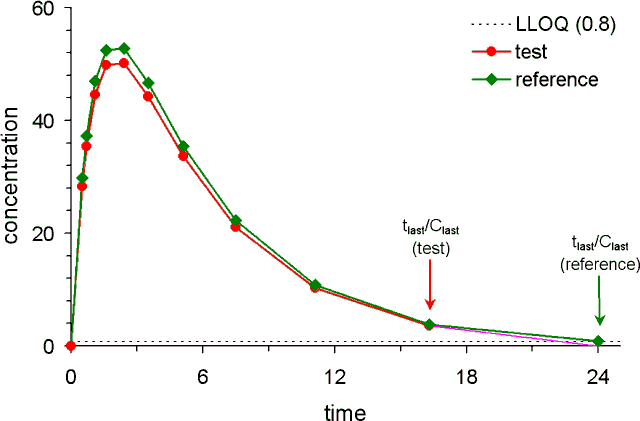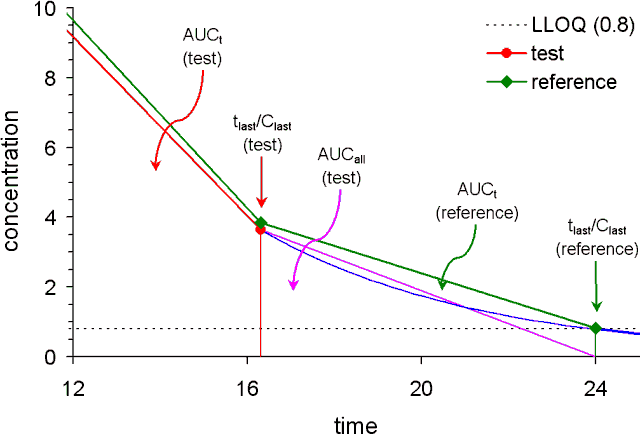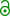## Calculation of AUCt, AUCall, AUCinf... [NCA / SHAM]

Hi Yu!

❝ Hi, its very nice to meet you.

❝ The informations from this forum are very helpful. I very appreciate it.

❝ how do you calculate the AUCt and do statistical analysis if there are concentrations below LOQ (at early or late sampling point)? As I know, the't' time in AUCt should be the same time. Someone recommend to make it zero in the early time. And they also recommend to delete it in the late time to calculate the AUCinf. Is it meaningful to do statistical analysis of AUCt with different 't' value? Please let me know how to proceed the study in such a case.

This topic comes up from time to time – not only on this forum, but also at the PKPD-list.

Let’s start with an example – a picture may tell more than a thousand words:
I generated data for a simple 1-compartment model, where ka = 1, kel = 0.2, and F×V/CL = 100 (reference) / 95 (test).
Since in BE we assume V and CL to be constant, and set Dtest = Dreference (in most regulations)
Frel = AUCtest / AUCreference.
AUC based on the parameters (calculating the definite integral) is 100/kel-100/ka for the reference and 95/kel-95/ka or AUCreference = 400 and AUCtest = 380. Therefore Frel = 380/400 = 95%. Fine.
Unfortunately we don’t have theoretical curves, but discrete sampling intervals, a lot of noise (biological and – to a much (!) lesser extent – analytical), and an artificial cut-off called LLOQ…
I used this algorithm (t1 0.5, tn 24, 10 sampling points in-between) to establish a sampling schedule. Sampling times were rounded to 2 decimal places, as well as the simulated concentrations; LLOQ was set to 0.8.
Obviously the concentration at the 24h sampling point was just above LLOQ for the reference (0.82), and BLQ (0.78) for the test.
┌──────┬──────┬──────┐ │  t   │ ref  │ test | ├──────┼──────┼──────┤ │ 0.00 │ 0.00 │ 0.00 | │ 0.50 │29.83 │28.34 | │ 0.71 │37.60 │35.72 | │ 1.01 │45.29 │43.02 | │ 1.44 │51.28 │48.72 | │ 2.05 │53.49 │50.82 | │ 2.91 │50.43 │47.91 | │ 4.14 │42.10 │39.99 | │ 5.89 │30.51 │28.99 | │ 8.37 │18.73 │17.79 | │11.90 │ 9.25 │ 8.79 | │16.92 │ 3.39 │ 3.22 | │24.00 │ 0.82 │(0.78)| └──────┴──────┴──────┘We can calculate the AUC by numerical integration up to 24h (tlast) for the reference, and only up to 16.92h (tlast) for the test…

An enlarged view of the the last section of the profile is given below:Now let’s start with the calculations (based on the linear trapezoidal rule for simplicity). We will assess the methods based on the bias (deviation from the theoretical value of T/R = 95%).

Method 1:
Calculation up to the last measured concentration for each formulation (AUCt)
┌───────────┬───────────┬────────┬────────┐ │   ref     │   test    │  T/R   │ % Bias │ ├───────────┼───────────┼────────┼────────┤ │ 401.88595 │ 367.64200 │ 91.48% │ -3.71% │ └───────────┴───────────┴────────┴────────┘

Method 2
Calculation up to the last time point where both formulations show concentrations above LLOQ (AUCp)
┌───────────┬───────────┬────────┬────────┐ │   ref     │   test    │  T/R   │ % Bias │ ├───────────┼───────────┼────────┼────────┤ │ 386.98255 │ 367.64200 │ 95.00% │  0.00% │ └───────────┴───────────┴────────┴────────┘

Method 3
Like Method #1, but a concentration of zero is assumed at the time point after tlast, i.e., a small triangle is added to AUCt. The method is available in WinNonlin; this metric was named by Pharsight ‘AUCall’ (you don’t find it in any textbook on PK – it’s Pharsight’s ‘invention’).
┌───────────┬───────────┬────────┬────────┐ │   ref     │   test    │  T/R   │ % Bias │ ├───────────┼───────────┼────────┼────────┤ │ 401.88595 │ 379.04080 │ 94.32% │ -0.72% │ └───────────┴───────────┴────────┴────────┘

Method 4
Extrapolation from tlast to t=∞ based on the observed concentration Clast (AUCinf, AUCinf-obs)
┌───────────┬───────────┬────────┬────────┐ │   ref     │   test    │  T/R   │ % Bias │ ├───────────┼───────────┼────────┼────────┤ │ 405.98047 │ 383.74829 │ 94.52% │ -0.50% │ └───────────┴───────────┴────────┴────────┘

Method 5
Extrapolation from tlast to t=∞ based on the predicted concentration Clast (AUCinf, AUCinf-pred)
┌───────────┬───────────┬────────┬────────┐ │   ref     │   test    │  T/R   │ % Bias │ ├───────────┼───────────┼────────┼────────┤ │ 405.93053 │ 383.74829 │ 94.54% │ -0.49% | └───────────┴───────────┴────────┴────────┘
(erroneous; see this post for corrections)

Now let’s look at the Pros and Cons.

Method #1 showed the largest bias, but has its merits. The method is available in all standard software (both commercial and open-source); the bias seen in the example most likely diminishes in ‘real world’ data sets. Pragmatically speaking: this method has already been applied in tenthousands of BE studies…

Although in this simple example Method #2 showed the smallest bias,1 I would not recommend it because:
• You would just throw away valuable information (all concentrations of the ‘longer’ profile after tlast of the ‘shorter’ profile will not be used).
• The method is not standard in any software I know. It would be rather tricky to get the right numbers out from a list of partial areas.
• You would make the life of inspectors miserable.
Method #3 is based on a flawed assumption. There’s only one concentration we simply know that it does not exist after a dose: Zero. Although it’s reasonable to start with a zero-concentration before the dose (the first triangle which is used in all methods), in some situations AUCall > AUCinf – which lead to a lot of questions in the past. This happens if a next sampling interval is quite large, and the estimated half life is comparable small. See also this post.

Extrapolation based on Method #4 is recommended by the FDA, but considered suboptimal (nicely speaking) by PK experts. Using this method we rely on the concentration with the largest variability (both inaccuracy and precision).
It’s definitely better to go with Method #5,2 which is standard according to the WHO, European, and many other countries’ guidelines.

Conclusion:
It’s like already discussed in this thread on sampling times; whatever method you choose, lay it down in the protocol and calculate all AUCs in the same manner. AUC is a quite robust metric, unless you have a relatively high LLOQ and large differences between formulations deviations simply mean out.
My personal recommendation would be AUCt calculated by the lin-up/log-down method followed by extrapolation by Method #5.

❝ Sorry for the poor english.

Oh, there are just a few native speakers here; we do all our best to communicate – somehow.

1. Edit (years later). Why not?
Fisher D, Kramer W, Burmeister Getz E. Evaluation of a Scenario in Which Estimates of Bioequivalence Are Biased and a Proposed Solution: tlast (Common). J Clin Pharm. 2016;56(7):794–800. doi:10.1002/jcph.663.free resource.
2. Method #5 is the default in EquivTest/PK (though #4 is available as well).

Dif-tor heh smusma 🖖🏼 Довге життя Україна!Helmut SchützThe quality of responses received is directly proportional to the quality of the question asked. 🚮
Science QuotesIng. Helmut Schütz